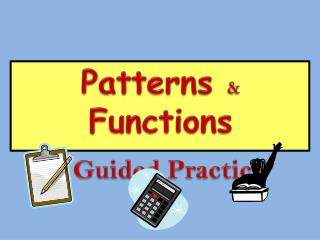Download PresentationPatterns & Functions Guided PracticePatterns & Functions Guided Practice - PowerPoint PPT Presentation

Download PresentationPatterns & Functions Guided Practice
An Image/Link below is provided (as is) to download presentation

Download Policy: Content on the Website is provided to you AS IS for your information and personal use and may not be sold / licensed / shared on other websites without getting consent from its author. While downloading, if for some reason you are not able to download a presentation, the publisher may have deleted the file from their server.

- - - - - - - - - - - - - - - - - - - - - - - - - - - E N D - - - - - - - - - - - - - - - - - - - - - - - - - - -
Presentation Transcript

1. Patterns & Functions Guided Practice

2. Teacher Notes • Students will need calculators and whiteboards with dry erase markers. • Paper and pencil can be used if whiteboards are not available. • Students should set up and attempt to solve the problems, and then check the accuracy of their work when the answers are shown on the screen.

3. The first step is to determine the rule for the pattern. Write the numbers in the pattern 2, 8, 14, 20, 26, 32

4. 2 8 14 20 26 32 +6 +6 +6 +6 +6 What could be the rule? Guess and Check

5. 32 38 44 50 56 62 2, 8, 14, 20, 26, 32 +6 +6 +6 +6 +6

6. The first six numbers in the pattern below were made using a subtraction rule. 85, 78, 71, 64, 57, 50 If the pattern continues the same way, what will be the next two numbers in the pattern? The answer for each problem is the same. Use subtractionto find the pattern.

7. The first six numbers in the pattern below were made using a subtraction rule. 85, 78, 71, 64, 57, 50 What will be the next two numbers in the pattern? Continue to subtract 7.

8. 12 8 3 • Multiply by 5 • Subtract 2 • Add 18 • Add 12 21 30 26

9. Karla is using a subtraction rule to make the number pattern shown below. 291, 288, 285, 282, 279 If the pattern continues in the same way, what will be the 7th number in the pattern? The answer for each problem is the same. Use subtractionto find the pattern. What is the pattern?

10. Karla is using a subtraction rule to make the number pattern shown below. 291, 288, 285, 282, 279 If the pattern continues in the same way, what will be the 7thnumber in the pattern? Continue subtracting 3. • 278 • 277 • 276 • 273 6th number in pattern 7thnumber in pattern

11. What is the rule? Function Chart A B C

12. Function Chart What is the rule? A B C

13. Function Chart What is the rule? A B C

14. Look at each pattern one at a time. What comes next for each part of the pattern? The next two items in this pattern are…

15. Figure 4 Figure 1 Figure 2 Figure 3 Figure 5 If this pattern continues, how many squares will be in the bottom row of Figure 8 of this pattern? Drawing the next few figures in the pattern will help solve this problem. • 7 • 8 • 10 • 12 Figure 7 Figure 6 Figure 8

16. Figure 4 Figure 1 Figure 2 Figure 3 Figure 5 If this pattern continues, how many squares will be in the TOP row of figure 7of this pattern? Drawing the next few figures in the pattern will help solve this problem. • 12 • 11 • 9 • 7 Figure 7 Figure 6

17. What is the rule? Function Chart A B C

18. What is the rule? Function Chart A B C

19. What is the rule? Function Chart A B C

20. First identify the rule.

21. First identify the rule. Guess and Check Does your rule work for every IN-OUT pair?

22. First identify the rule. 12 8 3 What number should come out if the number 7 goes into this number machine. 30 21 26 A. 18 C. 27 D. 25 D. 32

23. First identify the rule. 12 8 3 Guess and Check Does your rule work for every IN-OUT pair? If not, guess and check again. 30 21 26 X X

24. First identify the rule. 12 8 3 Guess and Check Does your rule work for every IN-OUT pair? 30 21 26

25. What number should come out if the number 7 goes into this number machine. A. 18 C. 27 D. 25 D. 32

26. Miller is buying packages of cookies for a party. Each package contains the same number of cookies. Based on the data in the table, how many packages will Miller need to buy to have a total of 42 cookies?

27. Based on the data in the table, how many packages will Miller need to buy to have a total of 42 cookies? Extend the Pattern

28. Look at each pattern one at a time. What comes next for each part of the pattern? The next two items in this pattern are…